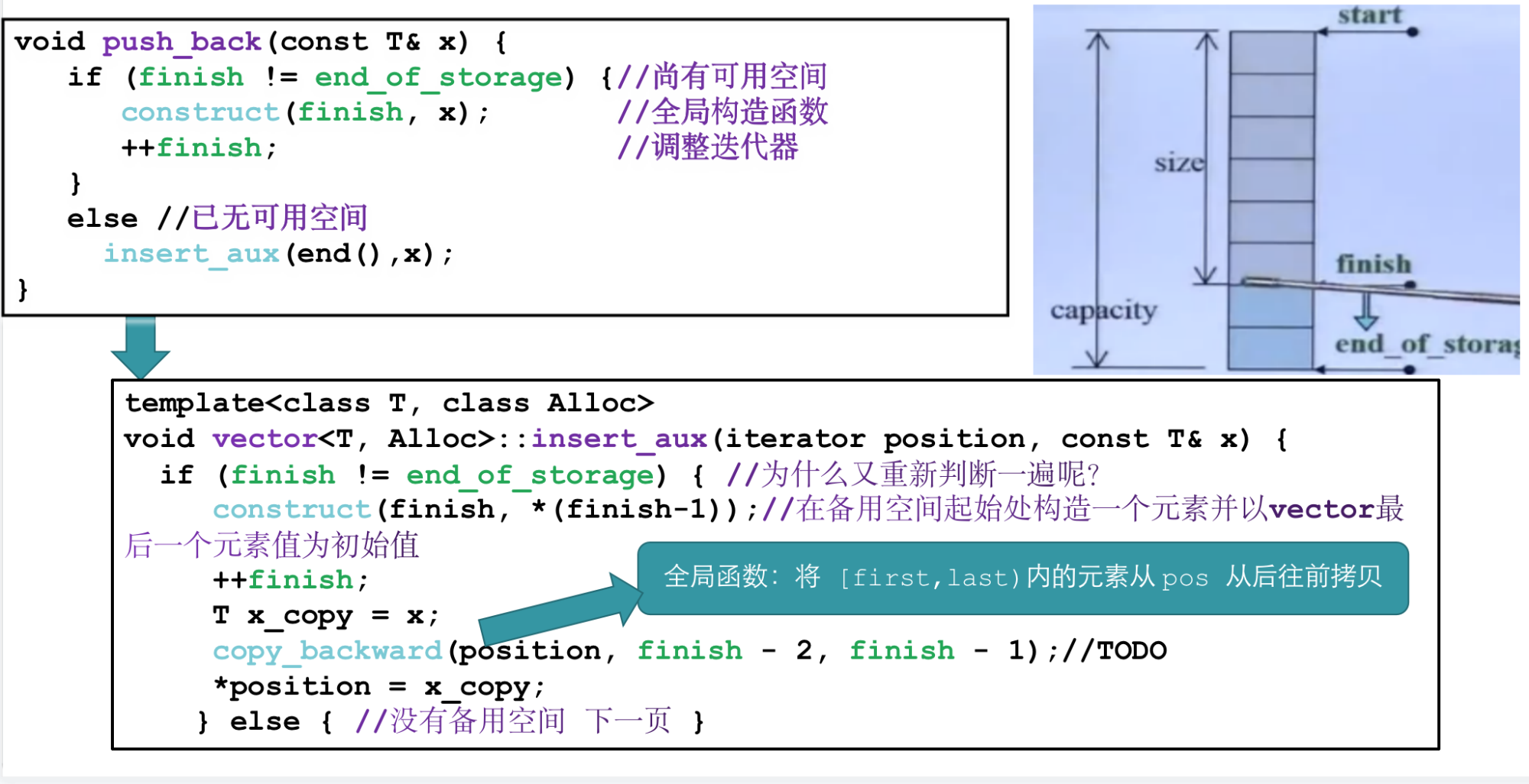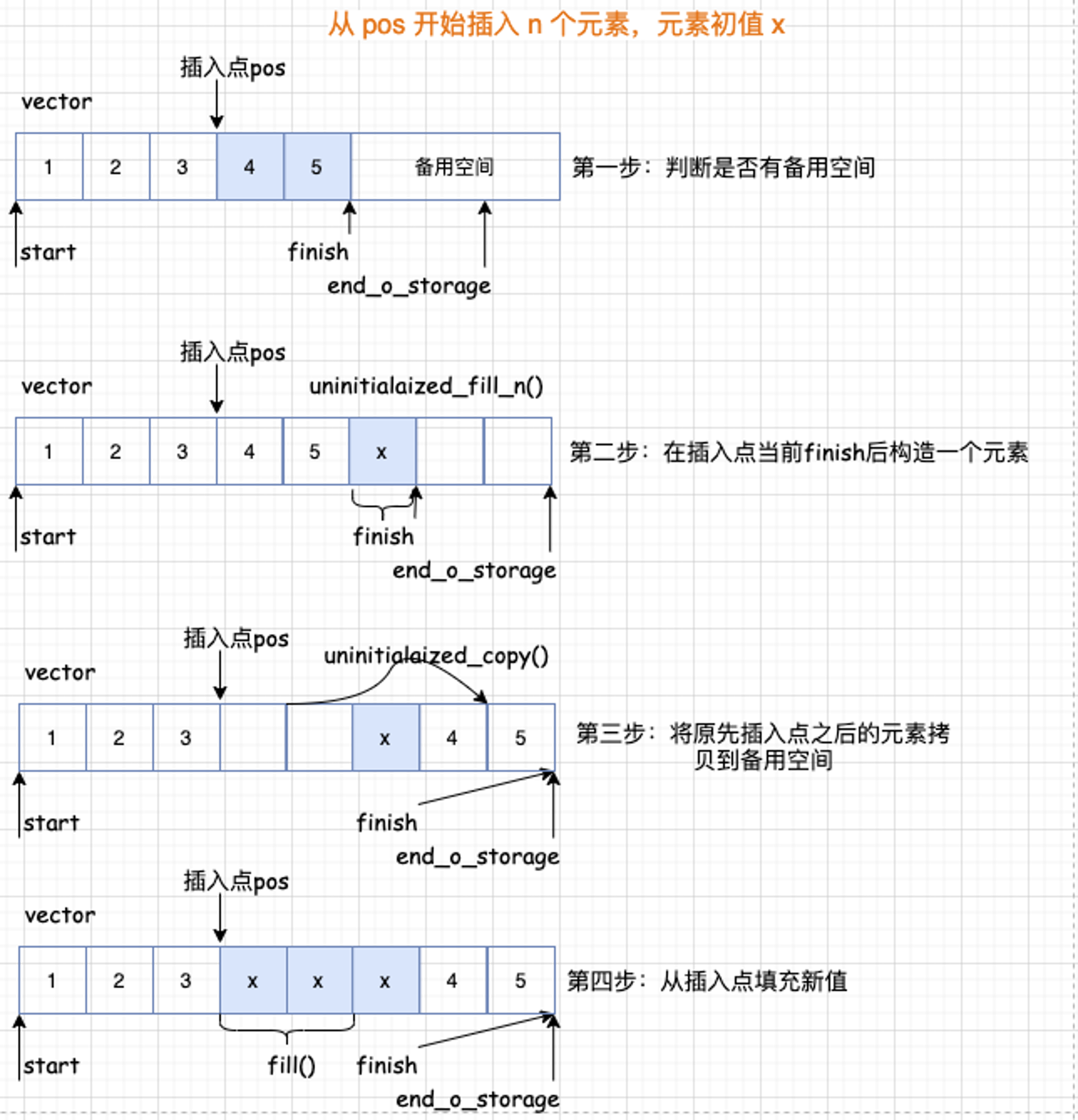墨滴herongwei

2021/03/26  阅读：21  主题：默认主题

# 超硬核 | 2 万字+20 图带你手撕 STL 序列式容器源码

## vector

#### vector 基本数据结构

• 迭代器，遍历容器的元素，控制容器空间的边界和元素的移动；
• 构造函数，满足容器的多种初始化；
• 属性的获取，比如 begin()，end()等；

vector 也不例外，其实看了源码之后就发现，vector 相反是所有容器里面最简单的一种。

``template <class T, class Alloc = alloc>class vector {public:   // 定义 vector 自身的嵌套型别    typedef T value_type;    typedef value_type* pointer;    typedef const value_type* const_pointer;    // 定义迭代器, 这里就只是一个普通的指针    typedef value_type* iterator;    typedef const value_type* const_iterator;    typedef value_type& reference;    typedef const value_type& const_reference;    typedef size_t size_type;    typedef ptrdiff_t difference_type;    ...  protected:    typedef simple_alloc<value_type, Alloc> data_allocator; // 设置其空间配置器    iterator start;    // 当前使用空间的头    iterator finish;   // 当前使用空间的尾    iterator end_of_storage; // 当前可用空间的尾    ...};``

#### 构造函数

vector 有多个构造函数, 为了满足多种初始化。

``void deallocate() { if (start)         data_allocator::deallocate(start, end_of_storage - start);}// 调用析构函数并释放内存~vector()  {  destroy(start, finish); deallocate();}``

#### 属性获取

``public: // 获取数据的开始以及结束位置的指针. 记住这里返回的是迭代器, 也就是 vector 迭代器就是该类型的指针.    iterator begin() { return start; }    iterator end() { return finish; }    reference front() { return *begin(); } // 获取值    reference back() { return *(end() - 1); }     const_iterator begin() const { return start; }// 获取右值    const_iterator end() const { return finish; }        const_reference front() const { return *begin(); }    const_reference back() const { return *(end() - 1); }        size_type size() const { return size_type(end() - begin()); }  // 数组元素的个数    size_type max_size() const { return size_type(-1) / sizeof(T); } // 最大能存储的元素个数    size_type capacity() const { return size_type(end_of_storage - begin()); } // 数组的实际大小    bool empty() const { return begin() == end(); }     //判断 vector 是否为空， 并不是比较元素为 0，是直接比较头尾指针。``

#### push 和 pop 操作

vector 的 push 和 pop 操作都只是对尾进行操作， 这里说的尾部是指数据的尾部。在上面这张图里，可以看到，push_back 这个函数里面又判断了一次 finish != end_of_storage 这是因为啥呢？原来这是因为 insert_aux 函数可能还被其他函数调用哦。

pop 元素

``public:  //将尾端元素拿掉 并调整大小  void pop_back() {      --finish;//将尾端标记往前移动一个位置 放弃尾端元素      destroy(finish);  }``

#### erase 删除元素

erase 函数清除指定位置的元素， 其重载函数用于清除一个范围内的所有元素。实际实现就是将删除元素后面所有元素往前移动，对于 vector 来说删除元素的操作开销还是很大的，所以说 vector 它不适合频繁的删除操作，毕竟它是一个数组。

``//清楚[first, last)中的所有元素  iterator erase(iterator first, iterator last) {      iterator i = copy(last, finish, first);      destroy(i, finish);      finish = finish - (last - first);      return first;  }  //清除指定位置的元素  iterator erase(iterator position) {      if (position + 1 != end())           copy(position + 1, finish, position);//copy 全局函数      }            --finish;      destroy(finish);      return position;  }  void clear() {      erase(begin(), end());  }``

##### insert 插入元素

vector 的插入元素具体来说呢，又分三种情况：

1、如果备用空间足够且插入点的现有元素多于新增元素；

2、如果备用空间足够且插入点的现有元素小于新增元素；

3、如果备用空间不够；

• 插入点之后的现有元素个数 > 新增元素个数
• 插入点之后的现有元素个数 <= 新增元素个数• 由于插入元素，使得容器元素整体迁移导致存放原容器元素的空间不再有效，从而使得指向原空间的迭代器失效；

• 由于删除元素，使得某些元素次序发生变化导致原本指向某元素的迭代器不再指向期望指向的元素。

• copy(a,b,c)：将(a,b)之间的元素拷贝到(c,c-(b-a))位置

• uninitialized_copy(first, last, result)： 具体作用是将 [first,last)内的元素拷贝到 result 从前往后拷贝

• copy_backward(first, last, result)： 将 [first,last)内的元素拷贝到 result 从后往前拷贝

#### vector 总结

• 在内存中分配一块连续的内存空间进行存，可以像数组一样操作，动态扩容。
• 随机访问方便，支持下标访问和vector.at()操作。
• 节省空间。

• 由于其顺序存储的特性，vector 插入删除操作的时间复杂度是 O(n)。
• 只能在末端进行pop和push。
• 当动态长度超过默认分配大小后，要整体重新分配、拷贝和释放空间。

vector的缺点也很明显, 在频率较高的插入和删除时效率就太低了

## list

list 的设计更加复杂一点，好处是每次插入或删除一个元素，就配置或释放一个元素，list 对于空间的运用有绝对的精准，一点也不浪费。而且对于任何位置的元素插入或删除，list 永远是常数空间。

#### list 数据结构-节点

__list_node 用来实现节点，数据结构中就储存前后指针和属性。

``template <class T> struct __list_node {    // 前后指针   typedef void* void_pointer;   void_pointer next;   void_pointer prev;    // 属性   T data;};``

``template<class T, class Ref, class Ptr> struct __list_iterator {   typedef __list_iterator<T, T&, T*>     iterator; // 迭代器   typedef __list_iterator<T, const T&, const T*> const_iterator;   typedef __list_iterator<T, Ref, Ptr>    self;       // 迭代器是bidirectional_iterator_tag类型   typedef bidirectional_iterator_tag iterator_category;   typedef T value_type;   typedef Ptr pointer;   typedef Ref reference;   typedef size_t size_type;   typedef ptrdiff_t difference_type;    ... };``

``template<class T, class Ref, class Ptr> struct __list_iterator {    ...    // 定义节点指针   typedef __list_node<T>* link_type;   link_type node; // 构造函数   __list_iterator(link_type x) : node(x) {}   __list_iterator() {}   __list_iterator(const iterator& x) : node(x.node) {}   ... };``

``template<class T, class Ref, class Ptr> struct __list_iterator  {    ... // 重载   bool operator==(const self& x) const { return node == x.node; }   bool operator!=(const self& x) const { return node != x.node; }    ...    // ++和--是直接操作的指针指向next还是prev, 因为list是一个双向链表   self& operator++() {      node = (link_type)((*node).next);     return *this;   }   self operator++(int) {      self tmp = *this;     ++*this;     return tmp;   }   self& operator--() {      node = (link_type)((*node).prev);     return *this;   }   self operator--(int)  {      self tmp = *this;     --*this;     return tmp;   }};``

#### list 结构

list 自己定义了嵌套类型满足 traits 编程， list 迭代器是 bidirectional_iterator_tag 类型，并不是一个普通指针。

list在定义 node 节点时， 定义的不是一个指针。这里要注意。

``template <class T, class Alloc = alloc>class list {protected:    typedef void* void_pointer;    typedef __list_node<T> list_node; // 节点    typedef simple_alloc<list_node, Alloc> list_node_allocator; // 空间配置器public:          // 定义嵌套类型    typedef T value_type;    typedef value_type* pointer;    typedef const value_type* const_pointer;    typedef value_type& reference;    typedef const value_type& const_reference;    typedef list_node* link_type;    typedef size_t size_type;    typedef ptrdiff_t difference_type;    protected:    // 定义一个节点, 这里节点并不是一个指针.    link_type node;    public:    // 定义迭代器    typedef __list_iterator<T, T&, T*>             iterator;    typedef __list_iterator<T, const T&, const T*> const_iterator; ...};``

#### list 构造和析构函数实现

list 默认使用 alloc 作为空间配置器，并根据这个另外定义了一个 list_node_allocator，目的是更加方便以节点大小来配置单元。

``template <class T, class Alloc = alloc>class list {protected:    typedef void* void_pointer;    typedef __list_node<T> list_node; // 节点    typedef simple_alloc<list_node, Alloc> list_node_allocator; // 空间配置器``

``class list {protected: // 配置一个节点并返回  link_type get_node() { return list_node_allocator::allocate(); }  // 释放一个节点  void put_node(link_type p) { list_node_allocator::deallocate(p); } // 产生(配置并构造)一个节点带有元素初始值  link_type create_node(const T& x) {      link_type p = get_node();      __STL_TRY {        construct(&p->data, x);      }      __STL_UNWIND(put_node(p));      return p;  }//销毁(析构并释放)一个节点  void destroy_node(link_type p) {    destroy(&p->data);    put_node(p);  }  // 对节点初始化  void empty_initialize() {     node = get_node();    node->next = node;    node->prev = node;  }  };``

#### 基本属性获取

``template <class T, class Alloc = alloc>class list {    ...public:  iterator begin() { return (link_type)((*node).next); } // 返回指向头的指针    const_iterator begin() const { return (link_type)((*node).next); }    iterator end() { return node; } // 返回最后一个元素的后一个的地址    const_iterator end() const { return node; }        // 这里是为旋转做准备, rbegin返回最后一个地址, rend返回第一个地址. 我们放在配接器里面分析    reverse_iterator rbegin() { return reverse_iterator(end()); }    const_reverse_iterator rbegin() const {       return const_reverse_iterator(end());     }    reverse_iterator rend() { return reverse_iterator(begin()); }    const_reverse_iterator rend() const {       return const_reverse_iterator(begin());    }         // 判断是否为空链表, 这是判断只有一个空node来表示链表为空.    bool empty() const { return node->next == node; }    // 因为这个链表, 地址并不连续, 所以要自己迭代计算链表的长度.    size_type size() const {      size_type result = 0;      distance(begin(), end(), result);      return result;    }    size_type max_size() const { return size_type(-1); }    // 返回第一个元素的值    reference front() { return *begin(); }    const_reference front() const { return *begin(); }    // 返回最后一个元素的值    reference back() { return *(--end()); }    const_reference back() const { return *(--end()); }        // 交换    void swap(list<T, Alloc>& x) { __STD::swap(node, x.node); }    ...};template <class T, class Alloc>inline void swap(list<T, Alloc>& x, list<T, Alloc>& y) {   x.swap(y);}``

#### list 的头插和尾插

``template <class T, class Alloc = alloc>class list {    ...    // 直接在头部或尾部插入    void push_front(const T& x) { insert(begin(), x); }     void push_back(const T& x) { insert(end(), x); }    // 直接在头部或尾部删除    void pop_front() { erase(begin()); }     void pop_back() {       iterator tmp = end();      erase(--tmp);    }    ...};``

``class list {    ...public:  // 最基本的insert操作, 之插入一个元素  iterator insert(iterator position, const T& x) {      // 将元素插入指定位置的前一个地址    link_type tmp = create_node(x);    tmp->next = position.node;    tmp->prev = position.node->prev;    (link_type(position.node->prev))->next = tmp;    position.node->prev = tmp;    return tmp;  }``

• 节点实际是以 node 空节点开始的。
• 插入操作是将元素插入到指定位置的前一个地址进行插入的。

#### 删除操作

``template <class T, class Alloc = alloc>class list {    ... iterator erase(iterator first, iterator last);    void clear();       // 参数是一个迭代器 修改该元素的前后指针指向再单独释放节点就行了 iterator erase(iterator position) {      link_type next_node = link_type(position.node->next);      link_type prev_node = link_type(position.node->prev);      prev_node->next = next_node;      next_node->prev = prev_node;      destroy_node(position.node);      return iterator(next_node);    }    ...};...}``

list 内部提供一种所谓的迁移操作(transfer)：将某连续范围的元素迁移到某个特定位置之前，技术上实现其实不难，就是节点之间的指针移动，只要明白了这个函数的原理，后面的 splice，sort，merge 函数也就一一知晓了，我们来看一下 transfer 的源码：

``template <class T, class Alloc = alloc>class list {    ...protected:    void transfer(iterator position, iterator first, iterator last) {      if (position != last) {        (*(link_type((*last.node).prev))).next = position.node;        (*(link_type((*first.node).prev))).next = last.node;        (*(link_type((*position.node).prev))).next = first.node;          link_type tmp = link_type((*position.node).prev);        (*position.node).prev = (*last.node).prev;        (*last.node).prev = (*first.node).prev;         (*first.node).prev = tmp;      }    }    ...};``

splice函数： 将两个链表进行合并：内部就是调用的 transfer 函数。

merge 函数： 将传入的 list 链表 x 与原链表按从小到大合并到原链表中(前提是两个链表都是已经从小到大排序了). 这里 merge 的核心就是 transfer 函数。

reverse 函数： 实现将链表翻转的功能：主要是 list 的迭代器基本不会改变的特点, 将每一个元素一个个插入到 begin 之前。

sort 函数： list 这个容器居然还自己实现一个排序，看一眼源码就发现其实内部调用的 merge 函数，用了一个数组链表用来存储 2^i 个元素, 当上一个元素存储满了之后继续往下一个链表存储, 最后将所有的链表进行 merge归并(合并), 从而实现了链表的排序。

• 原链表大 : 复制完后要删除掉原链表多余的元素
• 原链表小 : 复制完后要还要将x链表的剩余元素以插入的方式插入到原链表中

resize 操作： 重新修改 list 的大小。

clear 操作： 清除所有节点

remove 操作： 清除指定值的元素

unique 操作： 清除数值相同的连续元素，注意只有“连续而相同的元素”，才会被移除剩一个。

#### list 总结

list 是一种双向链表。每个结点都包含一个数据域、一个前驱指针 prev 和一个后驱指针 next。

• 不适用连续内存完成动态操作
• 在内部方便进行插入删除操作。
• 可在两端进行push和pop操作。

• 不支持随机访问，即下标操作和.at()。
• 相对于vector占用内存多。

## deque

### deque 的中控器

deque 在逻辑上看起来是连续空间，内部是由一段一段的定量连续空间构成。 一旦有必要在 deque 的前端或尾端增加新空间，便配置一段定量的连续空间，串接在整个 deque 的头部或尾部。

deque 采用一块所谓的 map （注意不是STL里面的map容器）作为中控器，其实就是一小块连续空间，其中的每个元素都是指针，指向另外一段较大的连续线性空间，称之为缓冲区。，在后面我们看到，缓冲区才是 deque 的储存空间主体。

``#ifndef __STL_NON_TYPE_TMPL_PARAM_BUGtemplate <class T, class Ref, class Ptr, size_t BufSiz>class deque {public:  typedef T value_type;  typedef value_type* pointer;  ...protected:  typedef pointer** map_pointer;  map_pointer map;//指向 map，map 是连续空间，其内的每个元素都是一个指针。  size_type map_size;  ...};``

### deque 的迭代器

deque 是分段连续空间，维持其“整体连续”假象的任务，就靠它的迭代器来实现，也就是 operator++ 和 operator-- 两个运算子上面。

``template <class T, class Ref, class Ptr, size_t BufSiz>struct __deque_iterator { // 迭代器定义  typedef __deque_iterator<T, T&, T*, BufSiz>             iterator;  typedef __deque_iterator<T, const T&, const T*, BufSiz> const_iterator;  static size_t buffer_size() {return __deque_buf_size(BufSiz, sizeof(T)); } // deque是random_access_iterator_tag类型  typedef random_access_iterator_tag iterator_category;  // 基本类型的定义, 满足traits编程  typedef T value_type;  typedef Ptr pointer;  typedef Ref reference;  typedef size_t size_type;  typedef ptrdiff_t difference_type;  // node  typedef T** map_pointer;  map_pointer node;  typedef __deque_iterator self;  ...};``

deque 的每一个缓冲区由设计了三个迭代器（为什么这样设计？）

``struct __deque_iterator { ...  typedef T value_type;  T* cur;  T* first;  T* last;  typedef T** map_pointer;  map_pointer node;  ...};``

first 表示当前数组中头的位置；

last 表示当前数组中尾的位置。

``inline size_t __deque_buf_size(size_t n, size_t sz) {  return n != 0 ? n : (sz < 512 ? size_t(512 / sz): size_t(1));}//如果 n 不为0，则返回 n，表示缓冲区大小由用户自定义//如果 n == 0，表示 缓冲区大小默认值//如果 sz = (元素大小 sizeof(value_type)) 小于 512 则返回 521/sz//如果 sz 不小于 512 则返回 1``

20 个元素需要 20/(sizeof(int)) = 3 个缓冲区。所以 map 运用了三个节点。迭代器 start 内的 cur 指针指向缓冲区的第一个元素，迭代器 finish 内的 cur 指针指向缓冲区的最后一个元素(的下一个位置)。

#### deque 迭代器的操作

operator++ 操作代表是需要切换到下一个元素，这里需要先切换再判断是否已经到达缓冲区的末尾。

``self& operator++() {   ++cur;      //切换至下一个元素  if (cur == last) {   //如果已经到达所在缓冲区的末尾 set_node(node+1);  //切换下一个节点 cur = first;    }  return *this;}``

operator-- 操作代表切换到上一个元素所在的位置，需要先判断是否到达缓冲区的头部，再后退。

``self& operator--() {       if (cur == first) {    //如果已经到达所在缓冲区的头部 set_node(node - 1); //切换前一个节点的最后一个元素 cur = last;    }  --cur;       //切换前一个元素  return *this;}``

#### deque 的构造和析构函数

``template <class T, class Alloc = alloc, size_t BufSiz = 0> class deque {    ...public:                         // Basic types  deque() : start(), finish(), map(0), map_size(0){    create_map_and_nodes(0);  }  // 默认构造函数  deque(const deque& x) : start(), finish(), map(0), map_size(0) {    create_map_and_nodes(x.size());    __STL_TRY {      uninitialized_copy(x.begin(), x.end(), start);    }    __STL_UNWIND(destroy_map_and_nodes());  }    // 接受 n:初始化大小, value:初始化的值  deque(size_type n, const value_type& value) : start(), finish(), map(0), map_size(0) {    fill_initialize(n, value);  }  deque(int n, const value_type& value) : start(), finish(), map(0), map_size(0) {    fill_initialize(n, value);  }   deque(long n, const value_type& value) : start(), finish(), map(0), map_size(0){    fill_initialize(n, value);  }  ...``

``void deque<T,Alloc,BufSize>::create_map_and_nodes(size_type_num_elements) {  //需要节点数= (每个元素/每个缓冲区可容纳的元素个数+1)  //如果刚好整除，多配一个节点  size_type num_nodes = num_elements / buffer_size() + 1;  //一个 map 要管理几个节点，最少 8 个，最多是需要节点数+2  map_size = max(initial_map_size(), num_nodes + 2);  map = map_allocator::allocate(map_size); // 计算出数组的头前面留出来的位置保存并在nstart.  map_pointer nstart = map + (map_size - num_nodes) / 2;  map_pointer nfinish = nstart + num_nodes - 1;  map_pointer cur;//指向所拥有的节点的最中央位置  ...}``

``// 如果 map 尾端的节点备用空间不足，符合条件就配置一个新的map(配置更大的，拷贝原来的，释放原来的)void reserve_map_at_back (size_type nodes_to_add = 1) {  if (nodes_to_add + 1 > map_size - (finish.node - map))    reallocate_map(nodes_to_add, false);}// 如果 map 前端的节点备用空间不足，符合条件就配置一个新的map(配置更大的，拷贝原来的，释放原来的)void reserve_map_at_front (size_type nodes_to_add = 1) {  if (nodes_to_add > start.node - map)    reallocate_map(nodes_to_add, true);}``

#### deque 的插入元素和删除元素

push 实现

``template <class T, class Alloc = alloc, size_t BufSiz = 0> class deque {    ...public:                         // push_* and pop_*    // 对尾进行插入    // 判断函数是否达到了数组尾部. 没有达到就直接进行插入  void push_back(const value_type& t) {    if (finish.cur != finish.last - 1) {      construct(finish.cur, t);      ++finish.cur;    }    else      push_back_aux(t);  }    // 对头进行插入    // 判断函数是否达到了数组头部. 没有达到就直接进行插入  void push_front(const value_type& t) {    if (start.cur != start.first) {      construct(start.cur - 1, t);      --start.cur;    }    else      push_front_aux(t);  }    ...};``

pop 实现

``template <class T, class Alloc = alloc, size_t BufSiz = 0> class deque {    ...public:     // 对尾部进行操作    // 判断是否达到数组的头部. 没有到达就直接释放    void pop_back() {    if (finish.cur != finish.first) {      --finish.cur;      destroy(finish.cur);    }    else      pop_back_aux();  }    // 对头部进行操作    // 判断是否达到数组的尾部. 没有到达就直接释放  void pop_front() {    if (start.cur != start.last - 1) {      destroy(start.cur);      ++start.cur;    }    else       pop_front_aux();  }    ...};``

reserve_map_at一类函数. pop和push都先调用了reserve_map_at_XX函数, 这些函数主要是为了判断前后空间是否足够.

#### 删除操作

deque 为了保证效率尽可能的高，就判断删除的位置是中间偏后还是中间偏前来进行移动。

``template <class T, class Alloc = alloc, size_t BufSiz = 0> class deque {    ...public:                         // Erase  iterator erase(iterator pos)   {    iterator next = pos;    ++next;    difference_type index = pos - start;      // 删除的地方是中间偏前, 移动前面的元素    if (index < (size() >> 1))     {      copy_backward(start, pos, next);      pop_front();    }      // 删除的地方是中间偏后, 移动后面的元素    else {      copy(next, finish, pos);      pop_back();    }    return start + index;  } // 范围删除, 实际也是调用上面的erase函数.  iterator erase(iterator first, iterator last);  void clear();     ...};``

deque 源码的基本每一个insert 重载函数都会调用了 insert_auto 判断插入的位置离头还是尾比较近。

fill_initialize 函数：：申请空间，对每个空间进行初始化，最后一个数组单独处理. 毕竟最后一个数组一般不是会全部填充满。

clear函数. 删除所有元素. 分两步执行: 首先从第二个数组开始到倒数第二个数组一次性全部删除，这样做是考虑到中间的数组肯定都是满的，前后两个数组就不一定是填充满的，最后删除前后两个数组的元素。

deque的swap操作也只是交换了start, finish, map, 并没有交换所有的元素.

resize函数. 重新将deque进行调整, 实现与list一样的.

#### deque 总结

deque 其实是在功能上合并了 vector 和 list。

1、随机访问方便，即支持 [ ] 操作符和 vector.at()；

2、在内部方便的进行插入和删除操作；

3、可在两端进行 push、pop

1、如果你需要高效的随即存取，而不在乎插入和删除的效率，使用 vector。

2、如果你需要大量的插入和删除，而不关心随机存取，则应使用 list。

3、如果你需要随机存取，而且关心两端数据的插入和删除，则应使用 deque 。

#### 以 deque 为底层容器的适配器

stack 的源码：

``#ifndef __STL_LIMITED_DEFAULT_TEMPLATEStemplate <class T, class Sequence = deque<T> >#elsetemplate <class T, class Sequence>#endifclass stack {public:  typedef typename Sequence::value_type value_type;  typedef typename Sequence::size_type size_type;  typedef typename Sequence::reference reference;  typedef typename Sequence::const_reference const_reference;protected:  Sequence c;``

queue 的源码：

``#ifndef __STL_LIMITED_DEFAULT_TEMPLATEStemplate <class T, class Sequence = deque<T> >#elsetemplate <class T, class Sequence>#endifclass queue {public:  typedef typename Sequence::value_type value_type;  typedef typename Sequence::size_type size_type;  typedef typename Sequence::reference reference;  typedef typename Sequence::const_reference const_reference;protected:  Sequence c;``

#### heap

push_heap 插入元素

``template <class RandomAccessIterator>inline void push_heap(RandomAccessIterator first, RandomAccessIterator last) {  __push_heap_aux(first, last, distance_type(first), value_type(first));}template <class RandomAccessIterator, class Distance, class T>inline void __push_heap_aux(RandomAccessIterator first, RandomAccessIterator last, Distance*, T*) {    // 这里传入的是两个迭代器的长度, 0, 还有最后一个数据  __push_heap(first, Distance((last - first) - 1), Distance(0),  T(*(last - 1)));}``

pop_heap 删除元素

pop操作其实并没有真正意义去删除数据, 而是将数据放在最后, 只是没有指向最后的元素而已, 这里arrary也可以使用, 毕竟没有对数组的大小进行调整. pop的实现有两种, 这里都罗列了出来, 另一个传入的是 cmp 伪函数.

``template <class RandomAccessIterator, class Compare>inline void pop_heap(RandomAccessIterator first, RandomAccessIterator last,                     Compare comp) {    __pop_heap_aux(first, last, value_type(first), comp);}template <class RandomAccessIterator, class T, class Compare>inline void __pop_heap_aux(RandomAccessIterator first,                           RandomAccessIterator last, T*, Compare comp) {  __pop_heap(first, last - 1, last - 1, T(*(last - 1)), comp,             distance_type(first));}template <class RandomAccessIterator, class T, class Compare, class Distance>inline void __pop_heap(RandomAccessIterator first, RandomAccessIterator last,                       RandomAccessIterator result, T value, Compare comp,                       Distance*) {  *result = *first;  __adjust_heap(first, Distance(0), Distance(last - first), value, comp);}template <class RandomAccessIterator, class T, class Distance>inline void __pop_heap(RandomAccessIterator first, RandomAccessIterator last,                       RandomAccessIterator result, T value, Distance*) {  *result = *first; // 因为这里是大根堆, 所以first的值就是最大值, 先将最大值保存.  __adjust_heap(first, Distance(0), Distance(last - first), value);}``

make_heap 将数组变成堆存放

``template <class RandomAccessIterator>inline void make_heap(RandomAccessIterator first, RandomAccessIterator last) {  __make_heap(first, last, value_type(first), distance_type(first));}template <class RandomAccessIterator, class T, class Distance>void __make_heap(RandomAccessIterator first, RandomAccessIterator last, T*,                 Distance*) {  if (last - first < 2) return;    // 计算长度, 并找出中间的根值  Distance len = last - first;  Distance parent = (len - 2)/2;      while (true) {      // 一个个进行调整, 放到后面    __adjust_heap(first, parent, len, T(*(first + parent)));    if (parent == 0) return;    parent--;  }}``

sort_heap 实现堆排序

``template <class RandomAccessIterator>void sort_heap(RandomAccessIterator first, RandomAccessIterator last) {  while (last - first > 1) pop_heap(first, last--);}template <class RandomAccessIterator, class Compare>void sort_heap(RandomAccessIterator first, RandomAccessIterator last,               Compare comp) {  while (last - first > 1) pop_heap(first, last--, comp);}``

#### priority_queue

priority_queue 因为也是队列的一种体现, 所以也就跟队列一样不能直接的遍历数组, 也就没有迭代器. priority_queue 本身也不算是一个容器, 它是以 vector 为容器以 heap为数据操作的配置器。

``#ifndef __STL_LIMITED_DEFAULT_TEMPLATEStemplate <class T, class Sequence = vector<T>,           class Compare = less<typename Sequence::value_type> >#elsetemplate <class T, class Sequence, class Compare>#endifclass  priority_queue {public: // 符合traits编程规范  typedef typename Sequence::value_type value_type;  typedef typename Sequence::size_type size_type;  typedef typename Sequence::reference reference;  typedef typename Sequence::const_reference const_reference;protected:  Sequence c; // 定义vector容器的对象  Compare comp; // 定义比较函数(伪函数)  ...};``

priority_queue 只有简单的 3 个属性获取的函数, 其本身的操作也很简单, 只是实现依赖了 vector 和 heap 就变得比较复杂。

``class  priority_queue { ...public:  bool empty() const { return c.empty(); }  size_type size() const { return c.size(); }  const_reference top() const { return c.front(); }    ...};``

push 和 pop 实现

push 和 pop 具体都是采用的 heap 算法。

priority_queue 本身实现是很复杂的，但是当我们已经了解过 vector，heap 之后再来看，它其实就简单了。

PS：看有多少人点赞，下期不定期更新关联式容器哦，先买个关子，下期有个硬核的内容带大家手撕红黑树源码，红黑树的应用可以说很广了，像 Java 集合中的 TreeSet 和 TreeMap、STL 中的 set 和 map、Linux 虚拟内存的管理都用到了哦。

1、《STL 源码剖析》

2、https://github.com/FunctionDou/STL

5 千字长文+ 30 张图解 | 陪你手撕 STL 空间配置器源码

## 更多精彩herongwei

2021/03/26  阅读：21  主题：默认主题